#Algebra 2 Worksheets

## Polynomial Functions Worksheets

Here is a graphic preview for all of the Polynomial Functions Worksheets. You can select different variables to customize these Polynomial Functions Worksheets for your needs. The Polynomial Functions Worksheets are randomly created and will never repeat so you have an endless supply of quality Polynomial Functions Worksheets to use in the classroom or at home. Our Polynomial Functions Worksheets are free to download, easy to use, and very flexible.

These Polynomial Functions Worksheets are a good resource for students in the 8th Grade through the 12th Grade.

Click here for a Detailed Description of all the Polynomial Functions Worksheets.

## Quick Link for All Polynomial Functions Worksheets

Click the image to be taken to that Polynomial Functions Worksheets.

##### Basic Polynomial OperationsPolynomial FunctionsWorksheets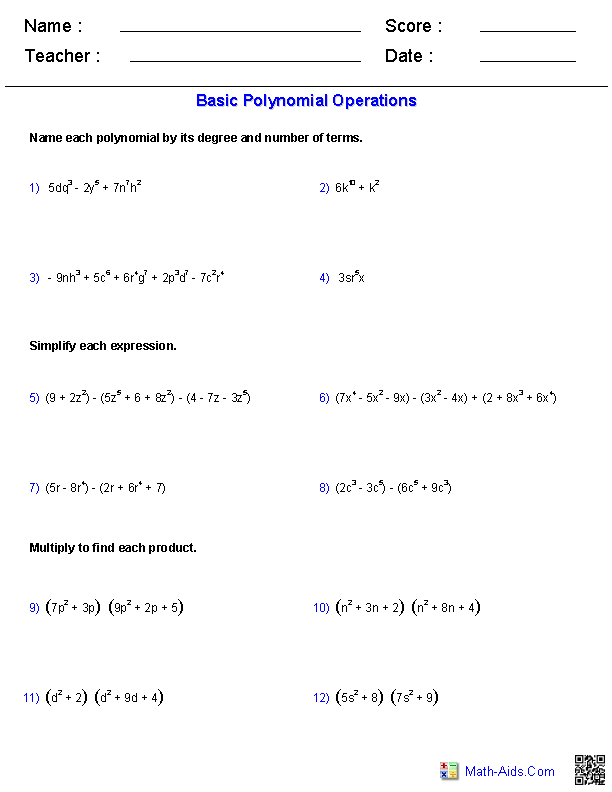##### FactoringSum/Difference of CubesPolynomial FunctionsWorksheets##### Factoring by GroupingPolynomial FunctionsWorksheets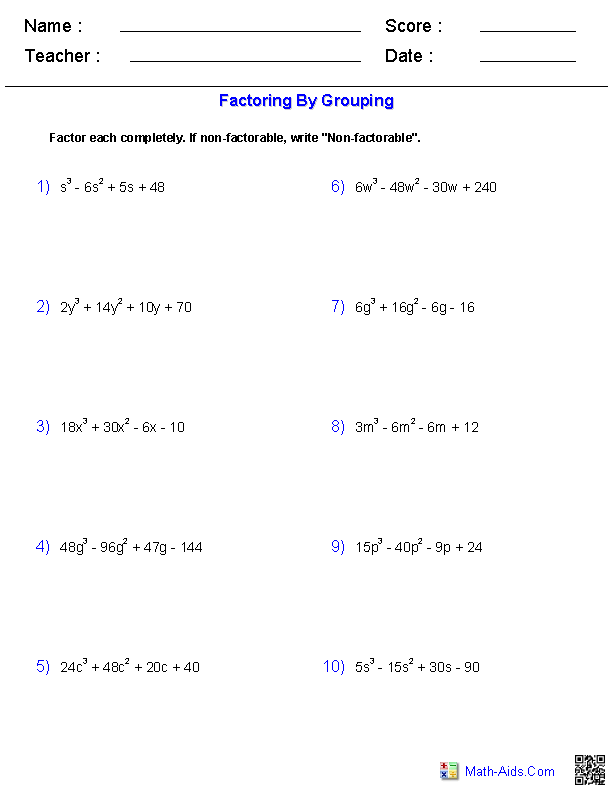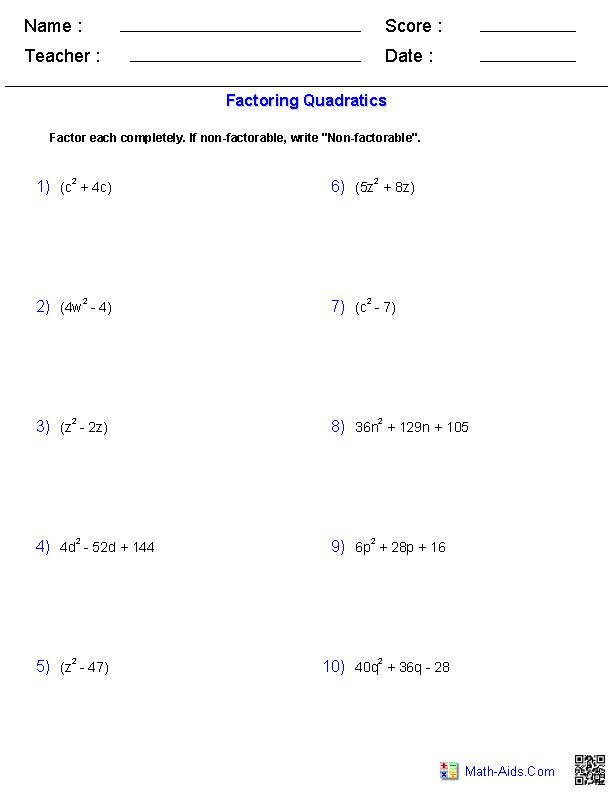##### Factoring All TechniquesPolynomial FunctionsWorksheets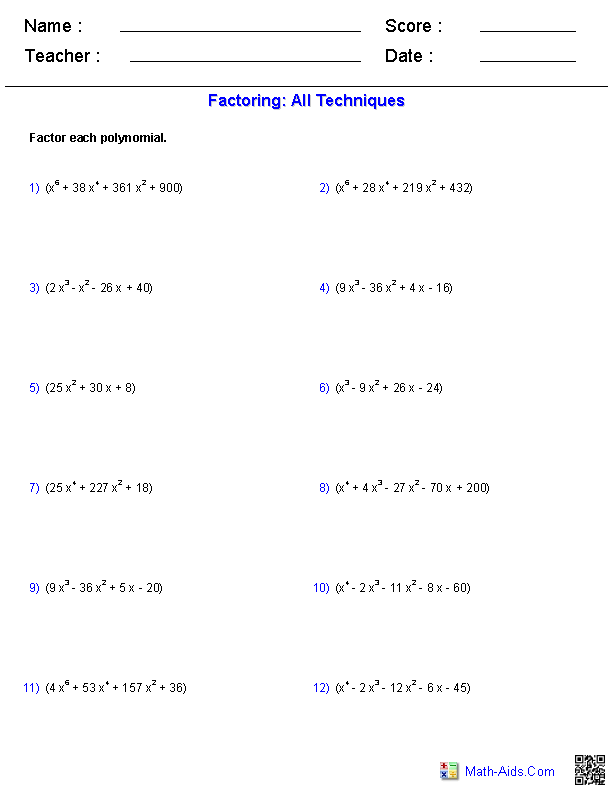##### Factors and ZerosPolynomial FunctionsWorksheets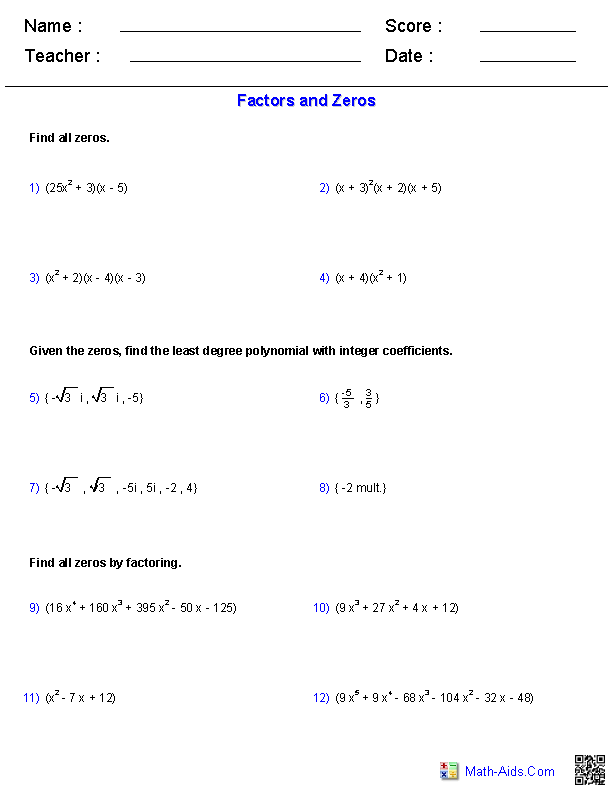##### The Remainder TheoremPolynomial FunctionsWorksheets##### Irrational and ImaginaryRoot TheoremsPolynomial FunctionsWorksheets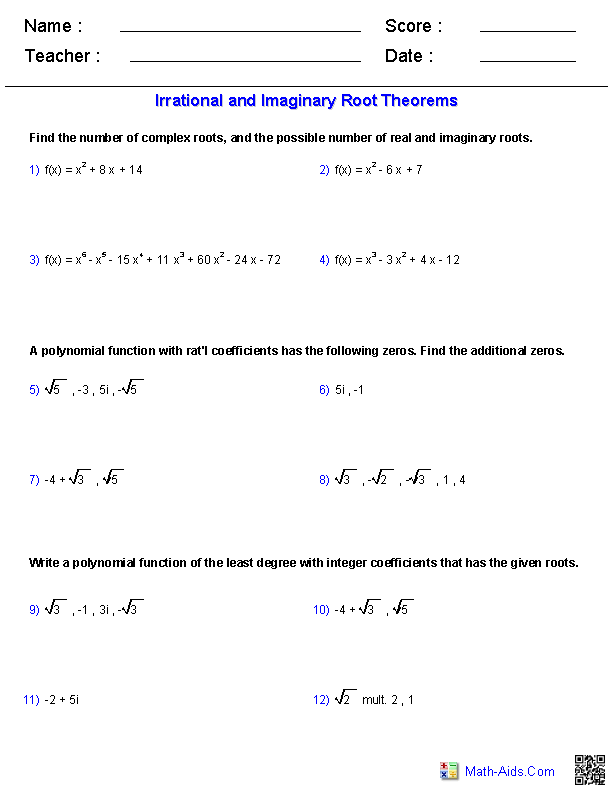##### Descartes' Rule of SignsPolynomial FunctionsWorksheets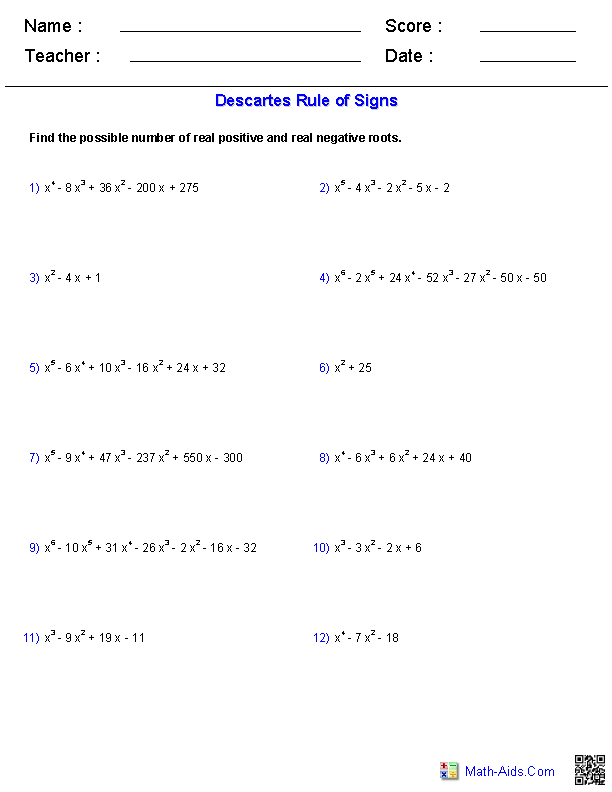##### The Rational Root TheoremPolynomial FunctionsWorksheets##### The Binomial TheoremPolynomial FunctionsWorksheets##### Analyzing and SolvingPolynomial EquationsWorksheets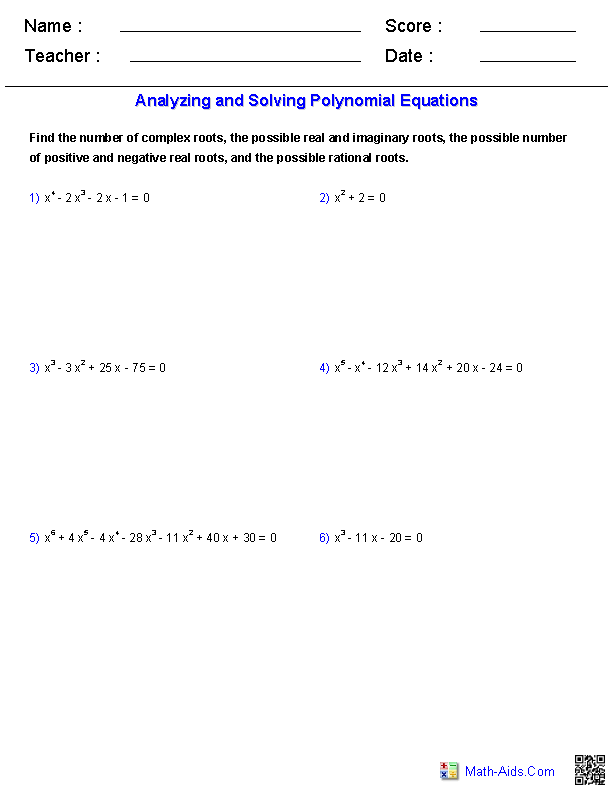##### Identify Graphs of Polynomial FunctionsWorksheets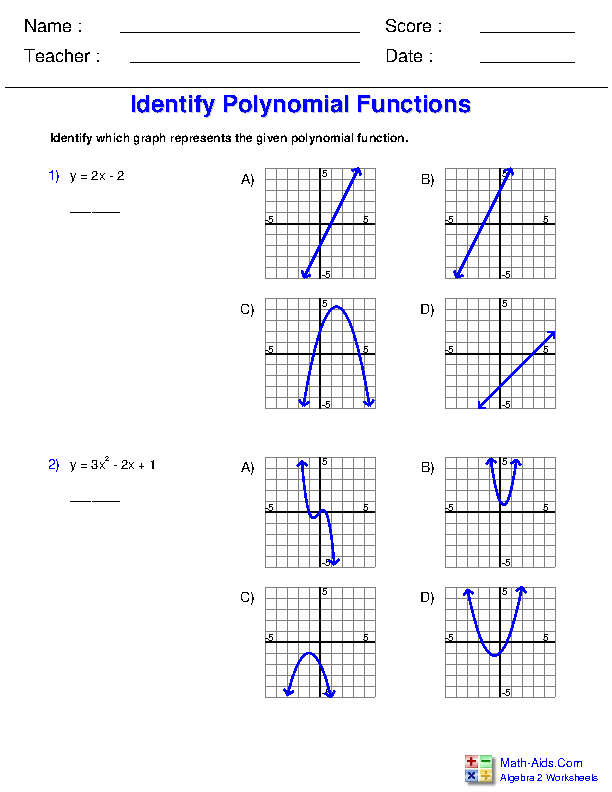Recommended Videos

## Description For All Polynomial Functions Worksheets

Basic Polynomial Operations Worksheets
This polynomial functions worksheet will produce problems for identifying the degree and term, simplify expressions, and finding the product for polynomials. You may select which type of polynomials problem to use. This polynomial worksheet will produce twelve problems per page. This polynomial worksheet is a good resource for students in the 9th Grade, 10th Grade, 11th Grade, and 12th Grade.

Factoring Sum/Differences of Cubes Worksheets
This polynomial functions worksheet will produce problems for factoring sum / differences of cubes. You may select the types of polynomials to factor and the coefficient of the first term. This polynomial worksheet will produce twelve problems per page. This polynomial worksheet is a good resource for students in the 9th Grade, 10th Grade, 11th Grade, and 12th Grade.

Factoring by Grouping Polynomial Worksheets
This monomial and polynomial worksheet will produce problems for factoring by grouping. You may select which type of monomials and polynomials problem to use. This monomial and polynomial worksheet will produce ten problems per page. This monomial and polynomial worksheet will produce ten problems per page. This monomials worksheet is a good resource for students in the 9th Grade, 10th Grade, 11th Grade, and 12th Grade.

This monomial and polynomial worksheet will produce problems for factoring quadratic polynomials. You may select which type of monomials and polynomials problem to use. This monomial and polynomial worksheet will produce ten problems per page. This monomial and polynomial worksheet will produce ten problems per page. This monomials worksheet is a good resource for students in the 9th Grade, 10th Grade, 11th Grade, and 12th Grade.

Factoring Using All Techniques Worksheets
This monomial and polynomial worksheet will produce problems for factoring polynomials using all techniques. You may select the degree of the polynomials problem to use. This monomial and polynomial worksheet will produce twelve problems per page. This monomial and polynomial worksheet will produce ten problems per page. This monomials worksheet is a good resource for students in the 9th Grade, 10th Grade, 11th Grade, and 12th Grade.

Factors and Zeros Worksheets
This monomial and polynomial worksheet will produce problems for factoring and finding zeros. You may select the degree of the polynomials and the type of zeros to find in the problems. This monomial and polynomial worksheet will produce twelve problems per page. This monomial and polynomial worksheet will produce ten problems per page. This monomials worksheet is a good resource for students in the 9th Grade, 10th Grade, 11th Grade, and 12th Grade.

The Remainder Theorem Worksheets
This monomial and polynomial worksheet will produce problems for working with the remainder theorem. You may select the degree of the polynomials to use in the problems. This monomial and polynomial worksheet will produce twelve problems per page. This monomial and polynomial worksheet will produce ten problems per page. This monomials worksheet is a good resource for students in the 9th Grade, 10th Grade, 11th Grade, and 12th Grade.

Irrational & Imaginary Root Theorems Worksheets
This monomial and polynomial worksheet will produce problems for working with irrational and imaginary root theorems. You may select the degree of the polynomials to use in the problems. This monomial and polynomial worksheet will produce twelve problems per page. This monomial and polynomial worksheet will produce ten problems per page. This monomials worksheet is a good resource for students in the 9th Grade, 10th Grade, 11th Grade, and 12th Grade.

Descartes' Rule of Signs Worksheets
This monomial and polynomial worksheet will produce problems for working with Descartes' Rule of Signs. You may select the degree of the polynomials to use in the problems. This monomial and polynomial worksheet will produce twelve problems per page. This monomial and polynomial worksheet will produce ten problems per page. This monomials worksheet is a good resource for students in the 9th Grade, 10th Grade, 11th Grade, and 12th Grade.

The Rational Root Theorem Worksheets
This monomial and polynomial worksheet will produce problems for working with The Rational Root Theorem. You may select the degree of the polynomials to use in the problems. This monomial and polynomial worksheet will produce twelve problems per page. This monomial and polynomial worksheet will produce ten problems per page. This monomials worksheet is a good resource for students in the 9th Grade, 10th Grade, 11th Grade, and 12th Grade.

The Binomial Theorem Worksheets
This monomial and polynomial worksheet will produce problems for working with The Binomial Theorem. You may select the degree of the polynomials to use in the problems. This monomial and polynomial worksheet will produce twelve problems per page. This monomial and polynomial worksheet will produce ten problems per page. This monomials worksheet is a good resource for students in the 9th Grade, 10th Grade, 11th Grade, and 12th Grade.

Analyzing & Solving Polynomial Equations Worksheets
This monomial and polynomial worksheet will produce problems for working with The Binomial Theorem. You may select the degree of the polynomials to use in the problems. This monomial and polynomial worksheet will produce twelve problems per page. This monomial and polynomial worksheet will produce six problems per page. This monomials worksheet is a good resource for students in the 9th Grade, 10th Grade, 11th Grade, and 12th Grade.

Identify Graphs of Polynomial Functions Worksheets
This monomial and polynomial worksheet will produce problems that ask students to identify graphs of polynomial functions. You may select the number of problems and types of polynomials to identify. This monomials and polynomials worksheet is a good resource for students in the 9th Grade, 10th Grade, 11th Grade, and 12th Grade.